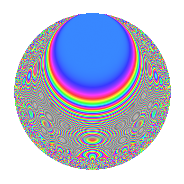# Properties

 Label 108.5.kLevel 108 Weight 5 Character orbit k Rep. character $$\chi_{108}(5,\cdot)$$ Character field $$\Q(\zeta_{18})$$ Dimension 72 Newform subspaces 1 Sturm bound 90 Trace bound 0

# Related objects

## Defining parameters

 Level: $$N$$ $$=$$ $$108 = 2^{2} \cdot 3^{3}$$ Weight: $$k$$ $$=$$ $$5$$ Character orbit: $$[\chi]$$ $$=$$ 108.k (of order $$18$$ and degree $$6$$) Character conductor: $$\operatorname{cond}(\chi)$$ $$=$$ $$27$$ Character field: $$\Q(\zeta_{18})$$ Newform subspaces: $$1$$ Sturm bound: $$90$$ Trace bound: $$0$$

## Dimensions

The following table gives the dimensions of various subspaces of $$M_{5}(108, [\chi])$$.

Total New Old
Modular forms 450 72 378
Cusp forms 414 72 342
Eisenstein series 36 0 36

## Trace form

 $$72q + 9q^{5} - 102q^{9} + O(q^{10})$$ $$72q + 9q^{5} - 102q^{9} + 18q^{11} - 225q^{15} - 282q^{21} - 1278q^{23} + 441q^{25} + 54q^{27} + 1854q^{29} - 1665q^{31} - 45q^{33} - 2673q^{35} + 6951q^{39} - 5472q^{41} + 1260q^{43} + 5553q^{45} + 5103q^{47} - 5904q^{49} + 1899q^{51} + 1107q^{57} - 10944q^{59} + 8352q^{61} - 11985q^{63} + 8757q^{65} + 378q^{67} + 5607q^{69} - 19764q^{71} + 6111q^{73} - 3453q^{75} - 5679q^{77} - 5652q^{79} - 20466q^{81} - 20061q^{83} + 26100q^{85} + 40545q^{87} + 15633q^{89} - 6039q^{91} + 40179q^{93} + 48024q^{95} - 37530q^{97} + 12177q^{99} + O(q^{100})$$

## Decomposition of $$S_{5}^{\mathrm{new}}(108, [\chi])$$ into newform subspaces

Label Dim. $$A$$ Field CM Traces $q$-expansion
$$a_2$$ $$a_3$$ $$a_5$$ $$a_7$$
108.5.k.a $$72$$ $$11.164$$ None $$0$$ $$0$$ $$9$$ $$0$$

## Decomposition of $$S_{5}^{\mathrm{old}}(108, [\chi])$$ into lower level spaces

$$S_{5}^{\mathrm{old}}(108, [\chi]) \cong$$ $$S_{5}^{\mathrm{new}}(27, [\chi])$$$$^{\oplus 3}$$$$\oplus$$$$S_{5}^{\mathrm{new}}(54, [\chi])$$$$^{\oplus 2}$$

## Hecke characteristic polynomials

There are no characteristic polynomials of Hecke operators in the database